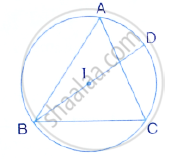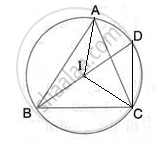Share

# In the Given Figure, I is the Incentre of δAbc. Bi When Produced Meets the Circumcircle of δAbc at D. ∠Bac = 55° and ∠Acb = 65°; Calculate: (I) ∠Dca, (Ii) ∠Dac, (Iii) ∠Dci, (Iv) Aic - Mathematics

Course

#### Question

In the given figure, I is the incentre of ΔABC. BI when produced meets the circumcircle of
ΔABC at D. ∠BAC = 55° and ∠ACB = 65°; calculate:
(i) ∠DCA, (ii) ∠DAC, (iii) ∠DCI, (iv) AIC#### SolutionJoin IA, IC and CD

(i) IB is the bisector of ∠ABC
⇒ ∠ABD = 1/2 ∠BC = 1/2 (180° - 65° - 55°) = 30°
∠DCA = ∠ABD = 30°
(Angle in the same segment)

(ii) ∠DAC = ∠CBD = 30°
(Angle in the same segment)

(iii) ∠ACI = ∠ACB = 65° = 32.5°
(CI is the angular bisector of ∠ACB )
∴ ∠DCI = ∠DCA + ∠ACI = 30° + 32.5° = 62.5°

(iv) ∠IAC = ∠BAC = 55° = 27.5°
(AI is the angular bisector of ∠BAC )
∴ ∠AIC = 180° - ∠IAC - ∠ICA = 180° - 27.5°- 32.5° = 120°

Is there an error in this question or solution?